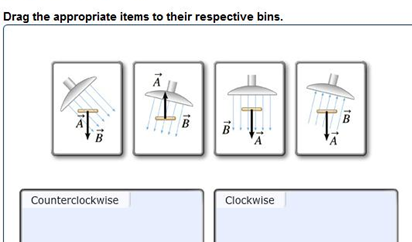# Problem: A metal detector uses a changing magnetic field to detect metallic objects. Suppose a metal detector that generates a uniform magnetic field perpendicular to its surface is held stationary at an angle of 15.0degree to the ground, while just below the surface there lies a silver bracelet consisting of 6 circular loops of radius 5.00 cm with the plane of the loops parallel to the ground. If the magnetic field increases at a constant rate of 0.0250 T/s, what is the induced emf, ε? Take the magnetic flux through an area to be positive when B crosses the area from top to bottom. Suppose you change either the orientation of B  or the direction of A so that the metal detector and the bracelet are arranged as shown in the diagrams below. Sort these situations based on whether the epsilon (or the current) induced in the bracelet by the increasing uniform magnetic field produced by the metal detector is in the counterclockwise or clockwise direction as viewed from above.

###### FREE Expert Solution
90% (393 ratings)View Complete Written Solution
###### Problem Details

A metal detector uses a changing magnetic field to detect metallic objects. Suppose a metal detector that generates a uniform magnetic field perpendicular to its surface is held stationary at an angle of 15.0degree to the ground, while just below the surface there lies a silver bracelet consisting of 6 circular loops of radius 5.00 cm with the plane of the loops parallel to the ground. If the magnetic field increases at a constant rate of 0.0250 T/s, what is the induced emf, ε? Take the magnetic flux through an area to be positive when B crosses the area from top to bottom. Suppose you change either the orientation of B  or the direction of A so that the metal detector and the bracelet are arranged as shown in the diagrams below. Sort these situations based on whether the epsilon (or the current) induced in the bracelet by the increasing uniform magnetic field produced by the metal detector is in the counterclockwise or clockwise direction as viewed from above.